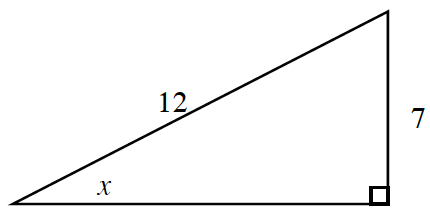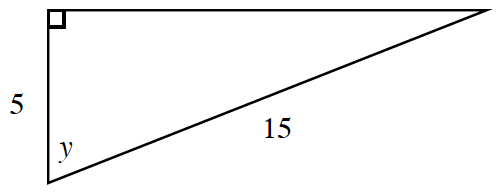### Home > PC3 > Chapter 1 > Lesson 1.2.2 > Problem1-88

1-88.

Use the idea of an inverse to calculate the measure of the indicated angle in each triangle.

1.Use appropriate trigonometric ratio.

$\text{sin}(x) = \frac{7}{12}$

Use the inverse sine button on a calculator.

$x\approx35.685°$

1.Follow the same procedure used in part (a).